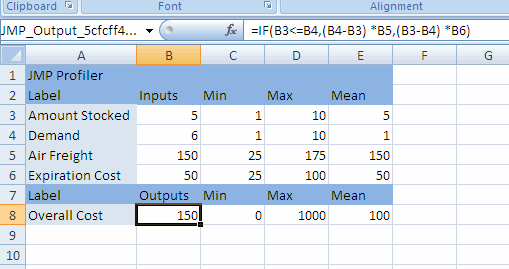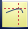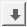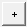Profilers > Excel Profiler > Example of an Excel Model
Publication date: 11/29/2021

# Example of an Excel Model

An Excel model consists of one or more Excel formulas. Each formula must be a function of one or more other cells. This example uses the Demand.xls sample import data located in the Samples/Import Data folder.

Figure 9.2 Demand Model in Excel#### About the Demand.xls Sample Import Data

The formula in cell B8 is a calculation of the Overall Cost associated with having different amounts of product in stock. The formula, which is shown in the Formula Bar, is a function of four cells:

Amount Stocked is the amount of product in stock.

Demand is the customer demand for the product.

Air Freight is the cost per unit to ship additional product by air when the demand exceeds the amount in stock.

Expiration Cost is the cost per unit of disposing of unused product when the demand is less than the amount in stock.

The calculations of the formula depend on the relationship between Amount Stocked and Demand.

If Amount Stocked is less than Demand, then the company has to ship additional units, at a cost of (Demand-Amount Stocked) × Air Freight. For example, if the demand is 8, but the company has only 6 in stock, then it has to ship 8 - 6 = 2 units at a cost of 2 × 150 = 300.

If Amount Stocked is greater than Demand, then the company has to dispose of unused product, at a cost of (Amount Stocked - Demand) × Expiration Cost. For example, if the demand is 5, but the company has 8 in stock, then it has to dispose of 8 - 5 = 3 units at a cost of 3 × 50 = 150.

If Amount Stocked is equal to Demand, then there is no shipping cost or disposal cost.

There is never both a shipping cost and a disposal cost at the same time.

#### Create the Model in Excel

1. Select Help > Sample Data Library and navigate up one level to the Samples/Import Data folder.

2. Double-click Demand.xls to open the file in Microsoft Excel.

3. In Microsoft Excel, click the JMP ribbon.

4. Click the Create/Edit Model button.

The name of the workbook is displayed in the Model and Model Name fields.

The Inputs and Outputs fields are populated with data from the worksheet.

5. Enter Customer Demand in the Model Name field and click Apply.

The Model field is updated.

6. Select Air Freight in the Inputs box and then click the down arrow button.

Air Freight is moved to the bottom of the list because you want it displayed last in the Profiler.

7. Click OK.

The Excel model is saved to the worksheet.

Notes:

If the fields in the Create/Edit Model window are not populated when you set up your model, click Choose and select the cell that contains the input or output name.

For inputs, specify the values and click Apply. Inputs must be values, not formulas.

For outputs, the specified cell must be a formula containing only information from the Input cells.

You can create more than one model for a worksheet. In the Create/Edit Model window, click the plus buttonnext to the Model name. In the Model Name field, change the name if necessary and click the Apply button. You can then change the inputs and outputs as necessary and click OK.

You must define the entire model on one worksheet. A model cannot reference cells on another worksheet.